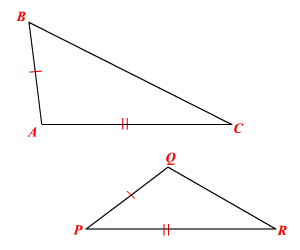# SSS Inequality

## SSS Inequality

If two sides of a triangle are congruent to two sides of another triangle and the third side of the first triangle is longer than the third side of the second, then the included angle of the first triangle is greater than the included angle of the second triangle.If $\stackrel{¯}{AB}\cong \stackrel{¯}{PQ},\text{\hspace{0.17em}}\text{\hspace{0.17em}}\stackrel{¯}{AC}\cong \stackrel{¯}{PR}$ and if $BC>QR$ , then $m\angle A>m\angle P$ .